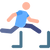top of page# Learning Objectives###### ​###### Jumping to Later Terms of APsIf we used the same thought process that we use for simple sequences, we would recall that sequences follow the below progression

a, a+d, a+2d, a+3d, a+4d, a+5d, …

Note that for T₂  the common difference, d had been added once, for T₃  it had been added twice, for T₄   it has been added three times, and so on.

Knowing this, we can conclude that arithmetic progressions have an nth term given by the rule

### Tₙ = a + (n-1)d

Where

• a = first term; and

• d = common difference.

This format of the rule is known as the nth term rule.###### ExampleSuppose that an AP has a 45th term of 180 and a 57th term of 228. Find

1. The 62nd term; and

2. The 1st term.

Solution:

Firstly, create a pair of simultaneous equations using the information given.

180 = a + 44d

228 = a + 56d

Now, solve the equations to find the value of d.

-48 = -12d

d = 4

Substitute the value of d into one of the original equations to find the value of a.

180 = a + 44(4)

a = 4.

We should now use this information to find solve the question.

1.  Find the 62nd term.

T₆₂ = 4 + (61)(4) = 248.

2. Find the first term.

T₁ = 4.###### ​###### ​###### ​###### ​###### ​###### ​bottom of page